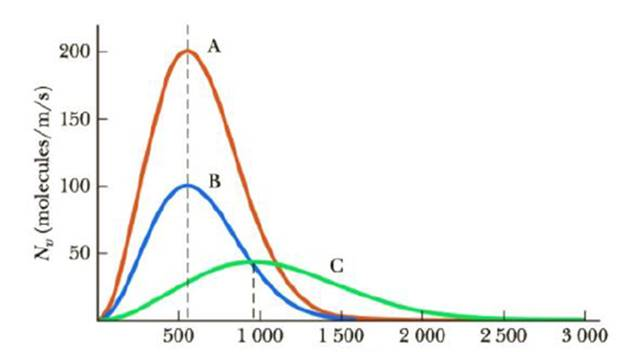Chapter 10, Problem 9CQ

Chapter
Section
Textbook Problem

Figure CQ10.9 shows Maxwell speed distributions for three different samples of oxygen (O2) gas. (a) Is the temperature of sample B greater than, less than, or equal to the temperature of sample A? (b) Is the temperature of sample C greater than, less than, or equal to the temperature of sample A?(a)

To determine
The temperature of sample B.

Explanation

The relation between temperature and rms speed is,

T=Mvrms23R

• M is the molar mass.
• R is the gas constant.
• T is the temperature

(b)

To determine
The temperature of sample C.

Still sussing out bartleby?

Check out a sample textbook solution.

See a sample solution

The Solution to Your Study Problems

Bartleby provides explanations to thousands of textbook problems written by our experts, many with advanced degrees!

Get Started